# How many moles of sodium hydroxide would have to be added to 125 mL of a...

How many moles of sodium hydroxide would have to be added to 125 mL of a 0.402 M hydrocyanic acid solution, in order to prepare a buffer with a pH of 9.500?

moles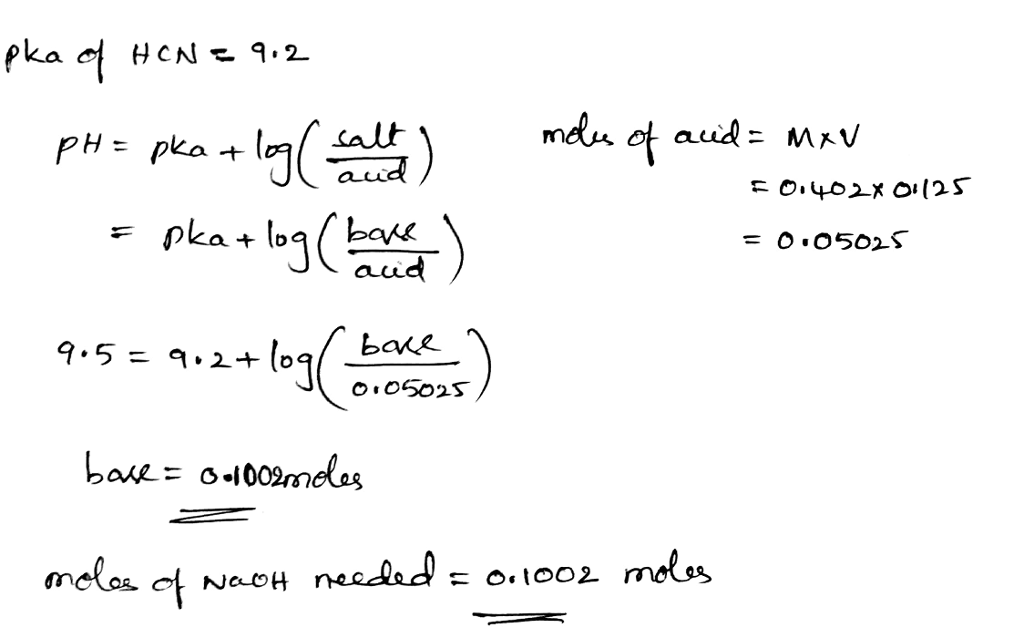##### Add Answer of: How many moles of sodium hydroxide would have to be added to 125 mL of a...
Similar Homework Help Questions
• ### How many moles of sodium hydroxide would have to be added to 225 mL of a 0.482 M nitrous acid sol...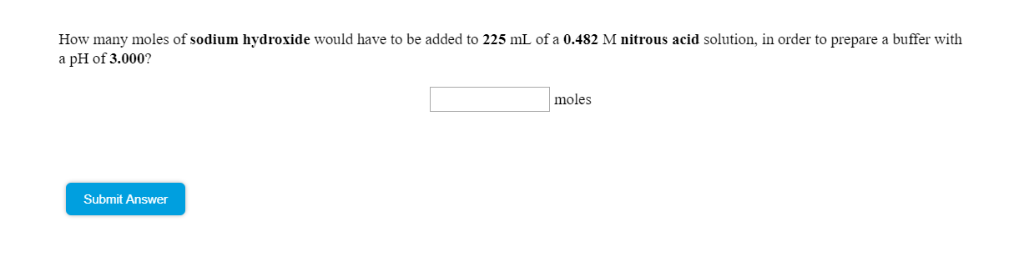How many moles of sodium hydroxide would have to be added to 225 mL of a 0.482 M nitrous acid solution, in order to prepare a buffer with a pH of 3.000? moles Submit Answer How many moles of sodium hydroxide would have to be added to 225 mL of a 0.482 M nitrous acid solution, in order to prepare a buffer with a pH of 3.000? moles Submit Answer

• ### qestOn How many moles of potassium hydroxide would have to be added to 225 mL of...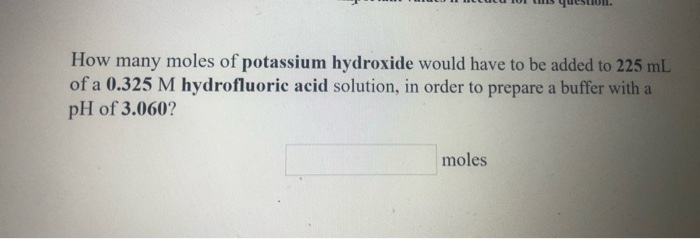qestOn How many moles of potassium hydroxide would have to be added to 225 mL of a 0.325 M hydrofluoric acid solution, in order to prepare a buffer with a pH of 3.060? moles An aqueous solution contains 0.428 M hypochlorous acid. How many mL of 0.292 M sodium hydroxide would have to be added to 150 mL of this solution in order to prepare a buffer witha pH of 7.140? mL

• ### NEL C UCUCU 01 LISUUS How many moles of sodium hydroxide would have to be added to 250 mL of a 0.350 M nitrous acid...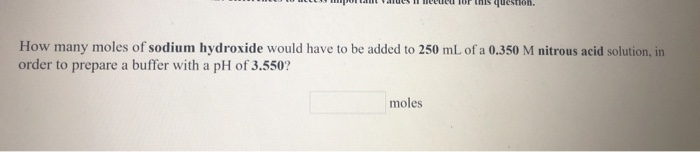NEL C UCUCU 01 LISUUS How many moles of sodium hydroxide would have to be added to 250 mL of a 0.350 M nitrous acid solution, in order to prepare a buffer with a pH of 3.550? moles

• ### Which of the following aqueous solutions are good buffer systems? . a. 0.15 M sodium hydroxide...

Which of the following aqueous solutions are good buffer systems? . a. 0.15 M sodium hydroxide + 0.29 M sodium bromide . b. 0.31 M hydrofluoric acid + 0.20 M potassium fluoride . c. 0.27 M nitric acid + 0.24 M potassium nitrate . d. 0.16 M hydrocyanic acid + 0.18 M potassium cyanide . e. 0.31 M ammonium nitrate + 0.30 M ammonia . b.How many moles of sodium hydroxide would have to be added to 250 mL of...

• ### How many moles of stontium hydroxide would react completely with

How many moles of stontium hydroxide would react completely with .500 mL of .40 M nitric acid?What happens to the pH of sodium carbonate as the barium chloride is added to it?

• ### References Use the References to access important values if needed for this question. An aqueous solution contains...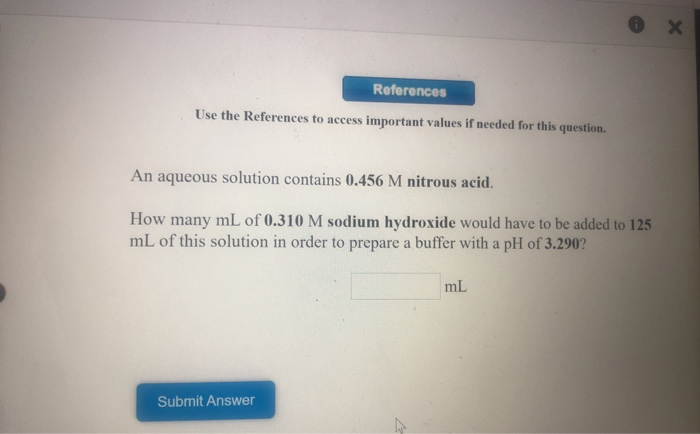References Use the References to access important values if needed for this question. An aqueous solution contains 0.456 M nitrous acid. How many mL of 0.3 10 M sodium hydroxide would have to be added to 125 mL of this solution in order to prepare a buffer with a pH of 3.290? mL Submit Answer References Use the References to access important values if needed for this question. solution contains 0.489 M ethylamine (C2H5NH2). An aqueous How many mL of...

• ### An aqueous solution contains 0.347 M methylamine (CH3NH2). How many mL of 0.212 M hydrobromic acid...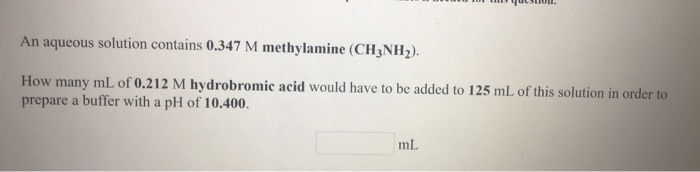An aqueous solution contains 0.347 M methylamine (CH3NH2). How many mL of 0.212 M hydrobromic acid would have to be added to 125 mL of this solution in order to prepare a buffer with a pH of 10.400. ml

• ### How many grams of solid sodium nitrite should be added to 0.500 L of a 0.114...

How many grams of solid sodium nitrite should be added to 0.500 L of a 0.114 M nitrous acid solution to prepare a buffer with a pH of 3.971 ? grams sodium nitrite = ------------ How many grams of solid ammonium chloride should be added to 0.500 L of a 0.134 M ammonia solution to prepare a buffer with a pH of 8.470 ? grams ammonium chloride = ------------ How many grams of solid sodium fluoride should be added to...

• ### 18. You are asked to prepare a pH = 8.60 buffer starting from 500 mL of...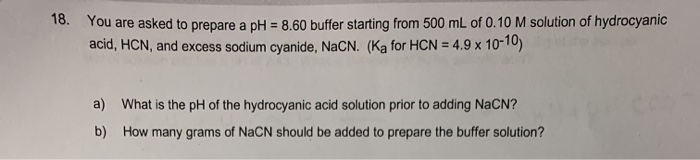18. You are asked to prepare a pH = 8.60 buffer starting from 500 mL of 0.10 M solution of hydrocyanic acid, HCN, and excess sodium cyanide, NACN. (Ka for HCN = 4.9 x 10-10) a) What is the pH of the hydrocyanic acid solution prior to adding NACN? b) How many grams of NaCN should be added to prepare the buffer solution?

• ### How many moles of sodium acetate must be added to 500.0 mL of 0250 Macetic acid...

How many moles of sodium acetate must be added to 500.0 mL of 0250 Macetic acid solution to produce a solution with a ph of 4.94? (The pK, of acetic acid is 4.74.) Select one O a. 0.021 moles b 013 moles C. 0.21 moles Dd. 0.20 moles O e 0011 moles

Need Online Homework Help?